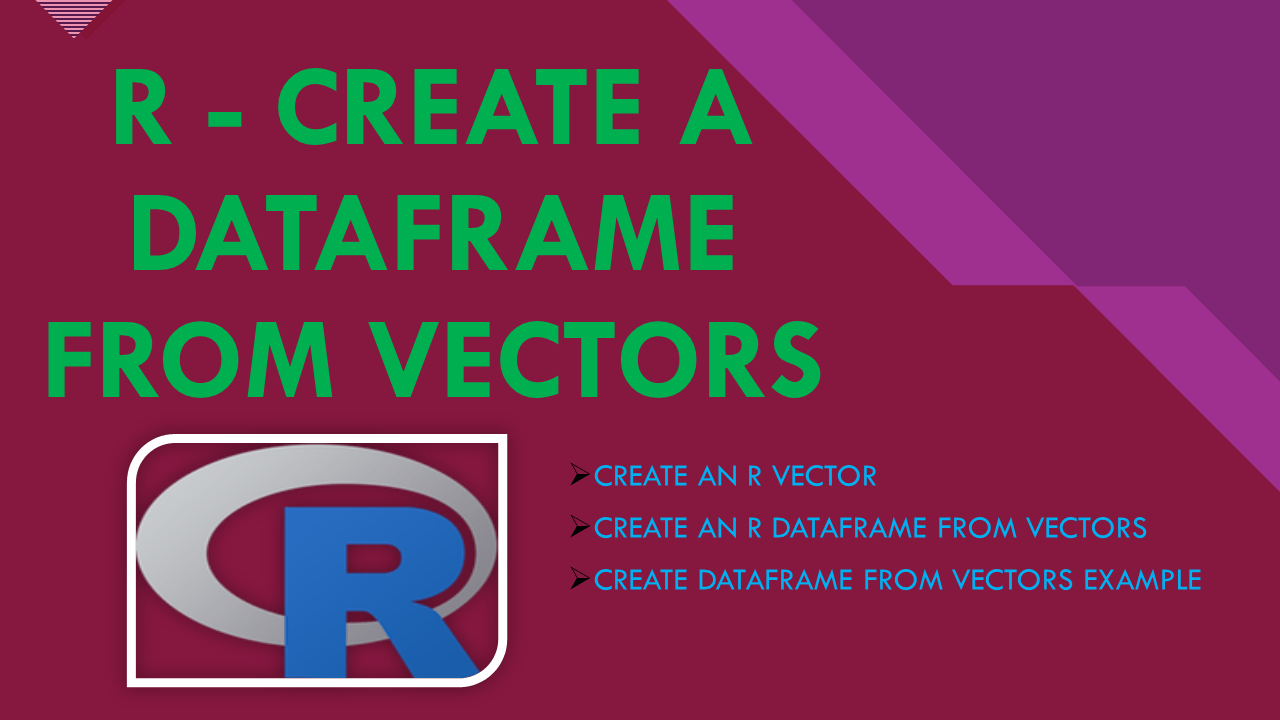# R – Create a DataFrame From Vectors

You can create a DataFrame from Vectors in R using `data.frame()`. In R, the Vector contains elements of the same type and the types can be logical, integer, double, character, complex or raw. You can create a Vector using `c()`. Whereas the R Data frame is a 2-dimensional structure that is used to hold the values in rows and columns. In the data frame, each column stores the values of one variable and each row stores the value of each column.

## 1. Create an R Vector

In R, Vector is a basic data structure that is used to store elements of the same data type. and the types can be logical, integer, double, character, complex or raw.

R Vector can be created by using c(). Let’s see syntax and create a vector.

``````
# Syntax of c() function
c(...)
``````

Now let’s create a Vector.

``````
# Create Vectors
id <- c(10,11,12,13)
name <- c('sai','ram','deepika','sahithi')
dob <- as.Date(c('1990-10-02','1981-3-24','1987-6-14','1985-8-16'))
``````

Here variables

• `id` – Numeric Vector which stores the numeric values.
• `name` – Character Vector which stores the character values.
• `dob` – Date Vector which stores the date values.

Let’s display the type of these Vector variables.

``````
# Types of Vectors
> typeof(id)
# "double"
> typeof(name)
# "character"
> typeof(dob)
# "double"
``````

## 2. Create an R DataFrame from Vectors

By using `data.frame()` function will create a DataFrame from Vector. A data frame is a list of variables of the same number of rows with unique row names. so all vectors you used to convert to DataFrame should have the same length, not having the same length will result in an error.

For syntax and usage of `data.frame()`, refer to How to Create a DataFrame in R.

You need to follow the below guidelines when creating a DataFrame from Vector in R using data.frame() function.

• The input objects passed to `data.frame()` should have the same number of rows.
• The column names should be non-empty.
• Duplicate column names are allowed, but you need to use `check.names = FALSE`.
• You can assign names to rows using `row.names` param.
• Character variables passed to `data.frame` are converted to factor columns.

### 2.1 Create DataFrame From Vectors Example

Now, let’s create a DataFrame from Vectors by using `data.frame()` function. This function takes the first argument either list or vector. I will use the Vectors that are created above and pass them as arguments to data.frame() to create a DataFrame from Vectors.

``````
# Create DataFrame
df <- data.frame(id,name,dob)

# Print DataFrame
df
``````

In the above example, I have used the following Vectors `id`, `name` and `dob` as arguments to the `data.frame()` function, separated by commas. The above example yields the below output. R will create a data frame with the column names/variables with the same names we used for Vector. You can use `df` or `print(df)` to print the DataFrame to the console.

``````
# Output
id    name        dob
1 10     sai 1990-10-02
2 11     ram 1981-03-24
3 12 deepika 1987-06-14
4 13 sahithi 1985-08-16
``````

Notice that it by default adds an incremental sequence number to each row in a DataFrame.

Alternatively, you can create a data.frame as follows by directly passing the vectors variables to the function.

``````
# Create DataFrame
df <- data.frame(
id = c(10,11,12,13),
name = c('sai','ram','deepika','sahithi'),
dob = as.Date(c('1990-10-02','1981-3-24','1987-6-14','1985-8-16'))
)

# Print DataFrame
df
``````

Let’s check the data types of the DataFrame by using `print(sapply(df, class))`. Note that I have not specified the data types of a column hence, R automatically infers the data type based on the data.

``````
# Display datatypes
print(sapply(df, class))

# Output
#         id        name         dob
#  "numeric"    "Factor"      "Date"
``````

You can also use `str(df)` to check the data types.

``````
# Display datatypes
str(df)

# Output
'data.frame':	4 obs. of  3 variables:
\$ id  : num  10 11 12 13
\$ name: Factor w/ 4 levels "deepika","ram",..: 4 2 1 3
\$ dob : Date, format: "1990-10-02" "1981-03-24" "1987-06-14" "1985-08-16"
``````

If you notice above the `name` column holds characters but its data type is Factor, by default, R always transforms character vectors to factors when creating a data frame with character vectors.

You can change this behavior by adding additional param `stringsAsFactors=False` while creating a DataFrame.

``````
# Create DataFrame
df <- data.frame(
id = c(10,11,12,13),
name = c('sai','ram','deepika','sahithi'),
dob = as.Date(c('1990-10-02','1981-3-24','1987-6-14','1985-8-16')),
stringsAsFactors=FALSE
)

# Print DataFrame
str(df)
``````

Yields below output. Note that the data type for the `name` column/variable is `chr` which is character. In R, you are often required to change the dataframe from `Factor` to `Charcter` before you perform some operations/transformations.

``````
# Output
'data.frame':	4 obs. of  3 variables:
\$ id  : num  10 11 12 13
\$ name: chr  "sai" "ram" "deepika" "sahithi"
\$ dob : Date, format: "1990-10-02" "1981-03-24" "1987-06-14" ...
``````

## 4. Complete Example

Following is a complete example of how to create a DataFrame from a Vectors in R or how to convert a Vector into a DataFrame.

``````
# Create R Vectors
id <- c(10,11,12,13)
name <- c('sai','ram','deepika','sahithi')
dob <- as.Date(c('1990-10-02','1981-3-24','1987-6-14','1985-8-16'))

# Check Vector types
typeof(id)
typeof(name)
typeof(dob)

# Create DataFrame
df <- data.frame(id,name,dob)
df

# Display DataFrame datatypes
print(sapply(df, class))
``````

## 5. Conclusion

In this article, you have learned how to create a Vector and convert Vectors to R DataFrame using examples. The Vector contains elements of the same type and the types can be logical, integer, double, character, complex or raw. You can create a Vector using `c()`. Whereas the R Data frame is a 2-dimensional structure that is used to hold the values in rows and columns.

## References

### NNK

SparkByExamples.com is a Big Data and Spark examples community page, all examples are simple and easy to understand and well tested in our development environment Read more ..